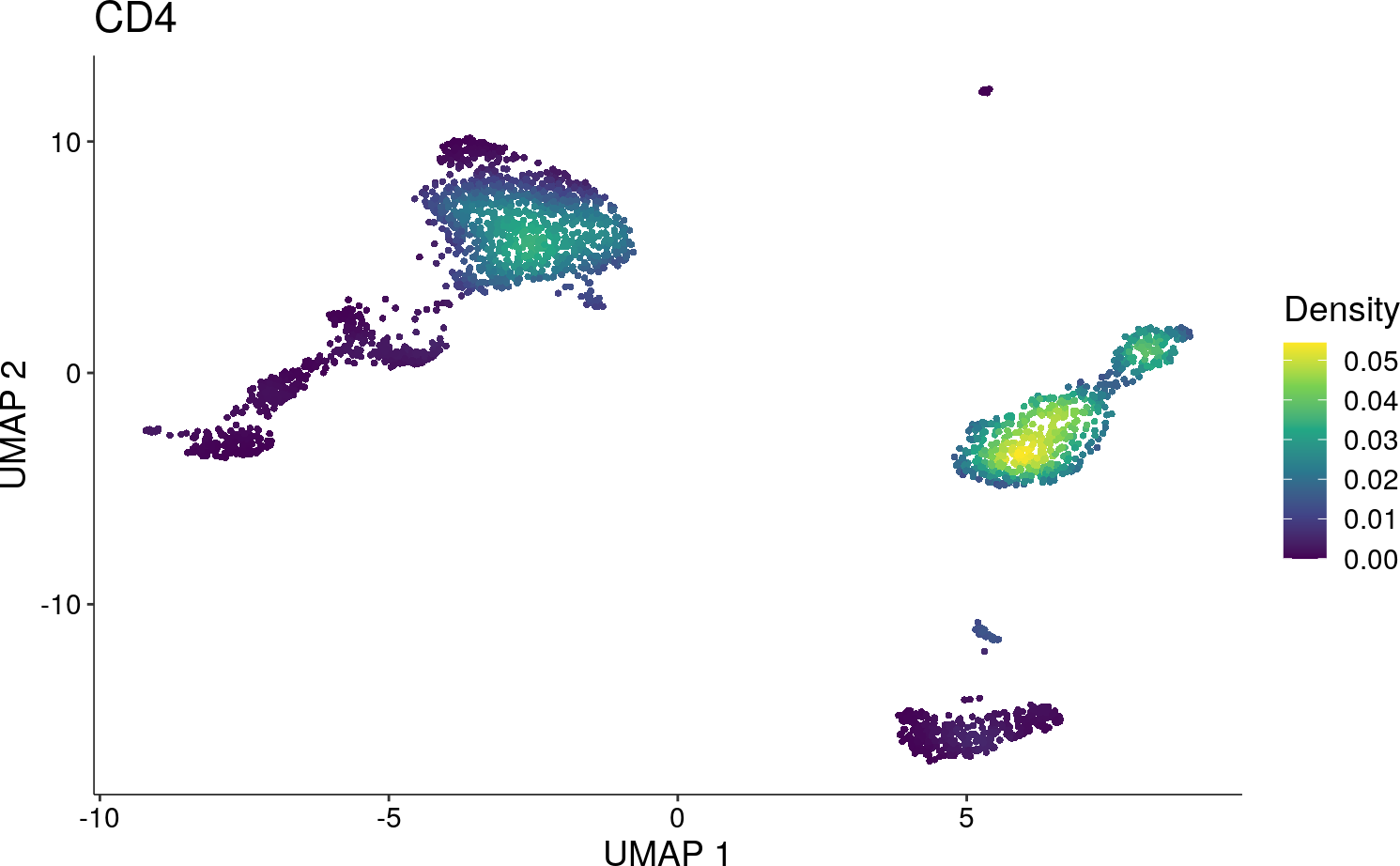# 1 Overview

Due to the sparsity observed in single-cell data (e.g. RNA-seq, ATAC-seq), the visualization of cell features (e.g. gene, peak) is frequently affected and unclear, especially when it is overlaid with clustering to annotate cell types. `Nebulosa` is an R package to visualize data from single cells based on kernel density estimation. It aims to recover the signal from dropped-out features by incorporating the similarity between cells allowing a “convolution” of the cell features.

# 2 Import libraries

For this vignette, let’s use `Nebulosa` with the `Seurat` package. First, we’ll do a brief/standard data processing.

``````library("Nebulosa")
library("Seurat")``````
``## Attaching SeuratObject``
``````##
## Attaching package: 'Seurat'``````
``````## The following object is masked from 'package:SummarizedExperiment':
##
##     Assays``````
``library("BiocFileCache")``

# 3 Data pre-processing

Let’s download a dataset of 3k PBMCs (available from 10X Genomics). This same dataset is commonly used in Seurat vignettes. The code below will download, store, and uncompress the data in a temporary directory.

``````bfc <- BiocFileCache(ask = FALSE)
data_file <- bfcrpath(bfc, file.path(
"https://s3-us-west-2.amazonaws.com/10x.files/samples/cell",
"pbmc3k",
"pbmc3k_filtered_gene_bc_matrices.tar.gz"
))

untar(data_file, exdir = tempdir())``````

Then, we can read the gene expression matrix using the `Read10X` from `Seurat`

``````data <- Read10X(data.dir = file.path(tempdir(),
"filtered_gene_bc_matrices",
"hg19"
))``````

Let’s create a Seurat object with features being expressed in at least 3 cells and cells expressing at least 200 genes.

``````pbmc <- CreateSeuratObject(
counts = data,
project = "pbmc3k",
min.cells = 3,
min.features = 200
)``````
``````## Warning: Feature names cannot have underscores ('_'), replacing with dashes
## ('-')``````

Remove outlier cells based on the number of genes being expressed in each cell (below 2500 genes) and expression of mitochondrial genes (below 5%).

``````pbmc[["percent.mt"]] <- PercentageFeatureSet(pbmc, pattern = "^MT-")
pbmc <- subset(pbmc, subset = nFeature_RNA < 2500 & percent.mt < 5)``````

## 3.1 Data normalization

Let’s use `SCTransform` to stabilize the variance of the data by regressing out the effect of the sequencing depth from each cell.

``pbmc <- SCTransform(pbmc, verbose = FALSE)``

## 3.2 Dimensionality reduction

Once the data is normalized and scaled, we can run a Principal Component Analysis (PCA) first to reduce the dimensions of our data from 26286 features to 50 principal components. To visualize the principal components, we can run a Uniform Manifold Approximation and Projection for Dimension Reduction (UMAP) using the first 30 principal components to obtain a two-dimentional space.

``````pbmc <- RunPCA(pbmc)
pbmc <- RunUMAP(pbmc, dims = 1:30)``````

## 3.3 Clustering

To assess cell similarity, let’s cluster the data by constructing a Shared Nearest Neighbor (SNN) Graph using the first 30 principal components and applying the Louvain algorithm.

``````pbmc <- FindNeighbors(pbmc, dims = 1:30)
pbmc <- FindClusters(pbmc)``````
``````## Modularity Optimizer version 1.3.0 by Ludo Waltman and Nees Jan van Eck
##
## Number of nodes: 2638
## Number of edges: 111648
##
## Running Louvain algorithm...
## Maximum modularity in 10 random starts: 0.8334
## Number of communities: 13
## Elapsed time: 0 seconds``````

# 4 Visualize data with `Nebulosa`

The main function from `Nebulosa` is the `plot_density`. For usability, it resembles the `FeaturePlot` function from `Seurat`.

Let’s plot the kernel density estimate for `CD4` as follows

``plot_density(pbmc, "CD4")``For comparison, let’s also plot a standard scatterplot using `Seurat`

``FeaturePlot(pbmc, "CD4")``